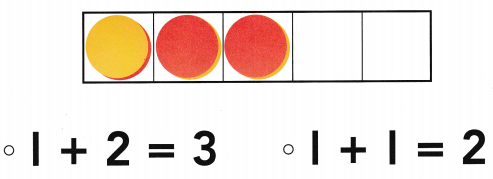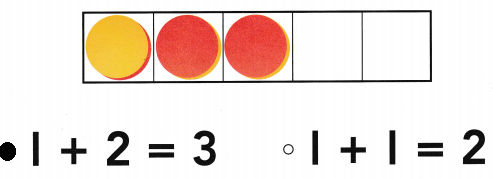# Texas Go Math Kindergarten Lesson 11.1 Answer Key Addition as Joining

Refer to our Texas Go Math Kindergarten Answer Key Pdf to score good marks in the exams. Test yourself by practicing the problems from Texas Go Math Kindergarten Lesson 11.1 Answer Key Addition as Joining.

## Texas Go Math Kindergarten Lesson 11.1 Answer Key Addition as Joining

Explore

DIRECTIONS: Listen to the addition word problem. Use counters. Trace the number that shows how many children are on the swings. Trace the plus symbol. Trace the number that shows how many children are being added to the group. Trace the is equal to symbol. Write the number that shows how many children there are now.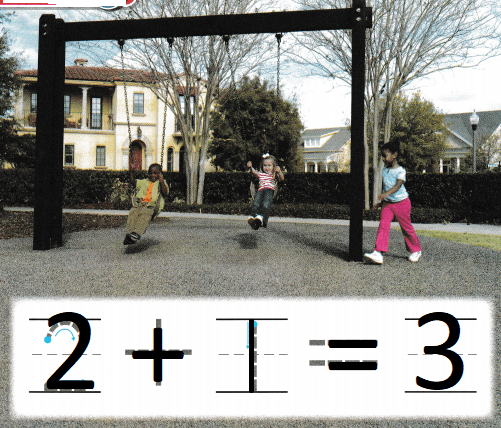Explanation:
Two children are on the swings.
and one more child is adding the group
so, 2 + 1 = 3
There are 3 children now.

Share and Show

DIRECTIONS: 1. Listen to the addition word problem. Use counters. Write the number that shows how many children are sitting. eating lunch. Trace the plus symbol and write the number that shows how many children are being added to the group. Trace the is equal to symbol and write the number that shows how many children are having lunch now.

Question 1.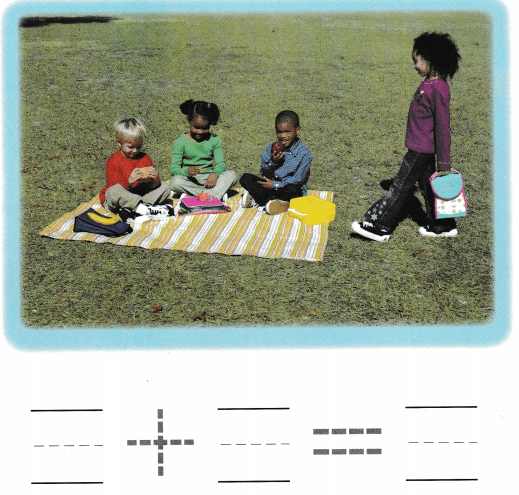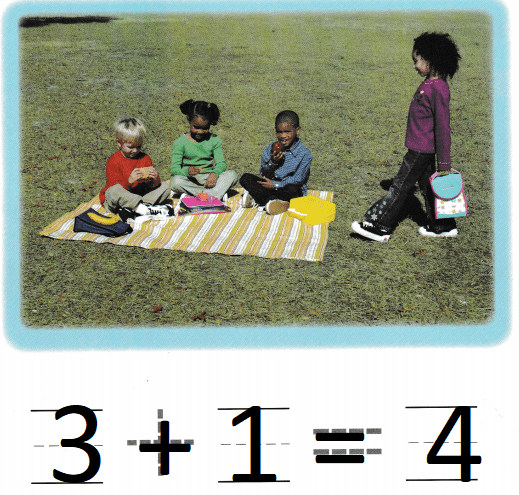Explanation:
3 children are sitting and eating lunch
one more child is going to attend
so. 3 + 1 = 4
There are 4 children in all.

DIRECTIONS: 2. Listen to the addition word problem. Use counters. Write the number that shows how many children are playing with the ball. Trace the plus symbol and write the number that shows how many children are being added to the group. Trace the is equal to symbol and write the number that shows how many children there are now.

Question 2.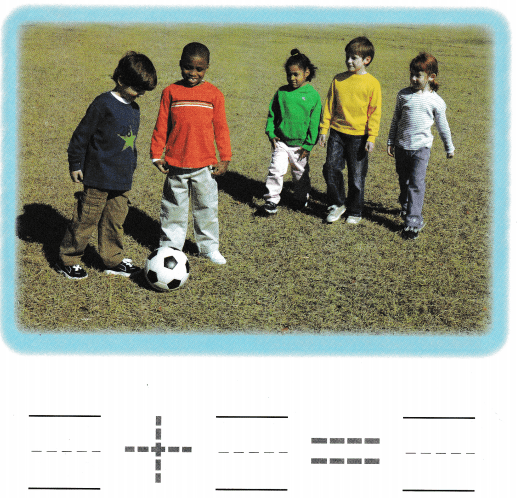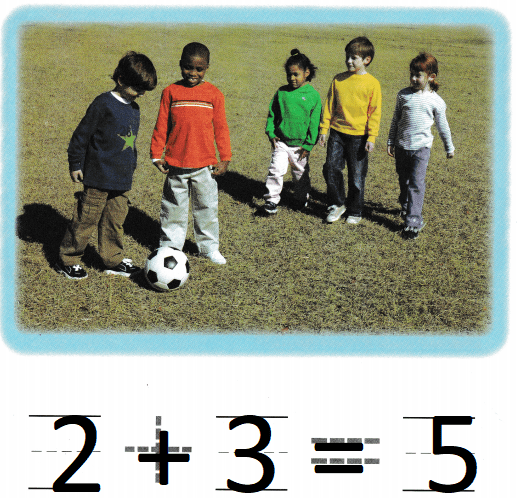Explanation:
2 children are playing with the ball
3 more children added to the group
so, there are 5 children in the group
2 + 3 = 5

HOME ACTIVITY • Show your child a set of four objects. Have him or her add one object to the set and tell how many there are now.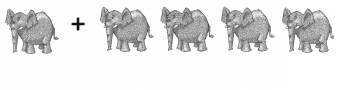Explanation:
There are 4 elephant toys
and one more is added to them
so, 4 + 1 = 5

DIRECTIONS: 3. Two sheep are in a pen. Then two sheep are added to the pen. How many sheep are there now? Draw to show the sheep. Write the numbers and trace the symbols to complete the number sentence. 4. Choose the correct answer. Which number sentence matches the model?

Problem Solving

Question 3.Explanation:
Two sheep are in a pen.
Then two sheep are added to the pen
2 + 2 = 4
so, There are 4 sheep in the pen.

Question 4.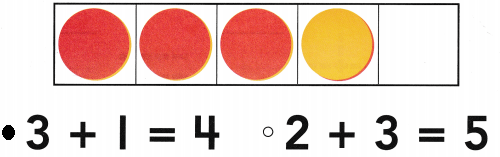Explanation:
In a five frame there are 3 red counters
and 1 yellow counter
3 + 1 = 4
so, there are 4 yellow counters.

### Texas Go Math Kindergarten Lesson 11.1 Homework and Practice Answer Key

DIRECTIONS: 1. Two children are playing with the sand. Two more children walk over to play. Write the number that shows how many children are playing. Write the number that shows how many children are being added to the group. Write the number that shows how many children there are now.

Question 1.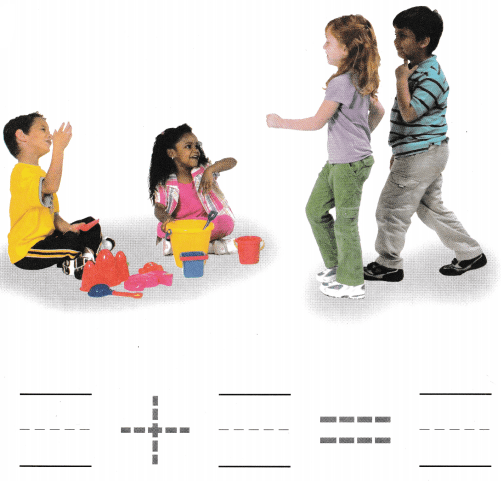Explanation:
Two children are playing with the sand.
Two more children walk over to play
2 + 2 = 4
so, there are 4 children in play

DIRECTIONS: Choose the correct answer. 2-3. Which number sentence matches the model?

Lesson Check

Question 2.Explanation:
In a five frame there are 2 red counters
and 3 yellow counters
2 + 3= 5
so, there are 5 yellow counters.

Question 3.